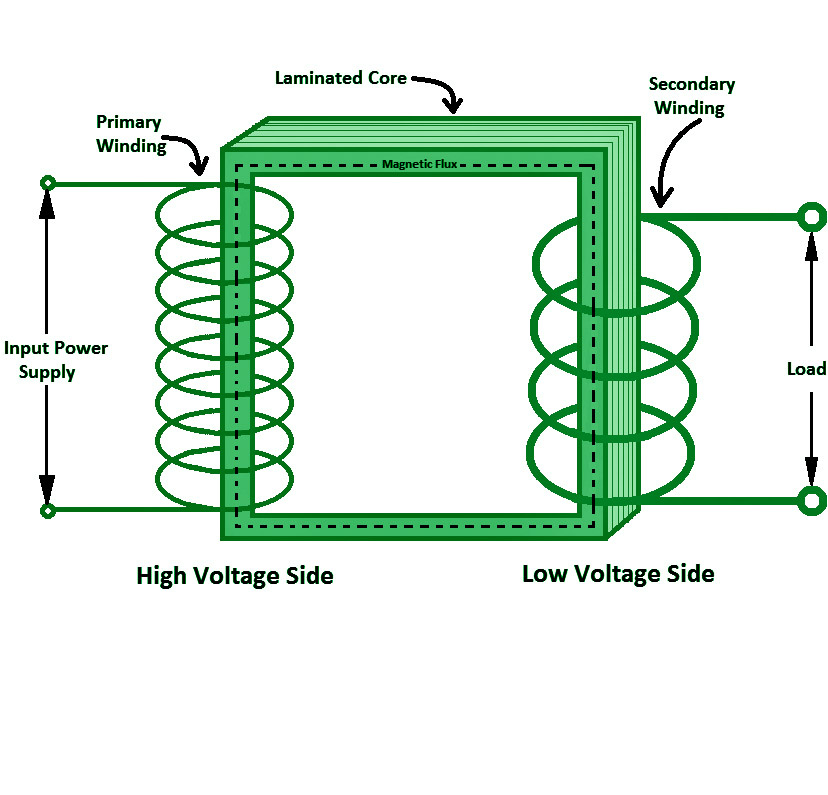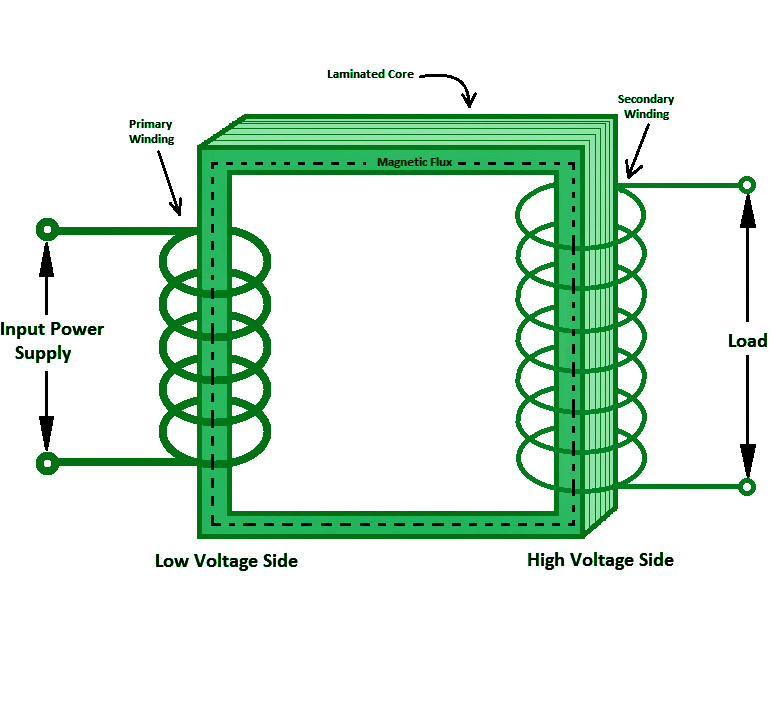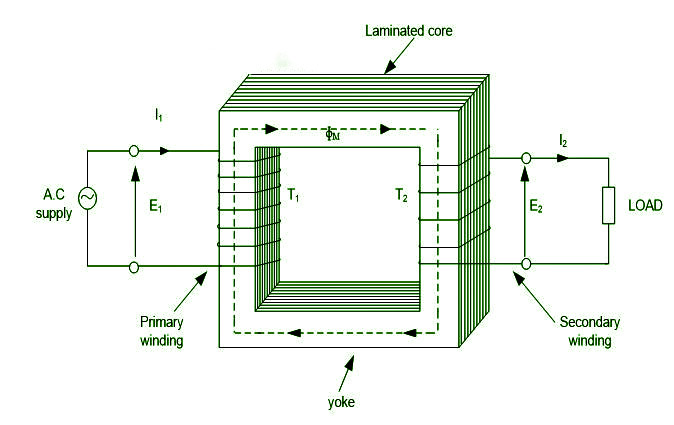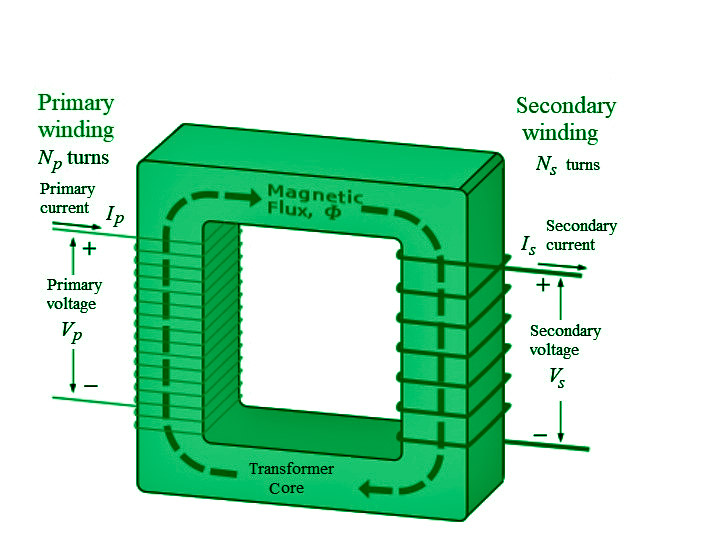# Transformers – Definition, Types, Working, Applications

• Last Updated : 02 Nov, 2021

The direct current (DC) travels in only one direction, but the alternating current (AC) is an electric current that switches direction on occasion and changes its amplitude continually over time. Alternating current is the type of electricity used by companies and consumers when they plug in kitchen appliances, TVs, fans, and electric lamps to a wall socket.

A flashlight’s battery cell, on the other hand, is a common DC power source. The abbreviations AC and DC are often used to signify alternating and direct current and voltage, respectively. The most common waveform of alternating current in most electric power circuits is a sine wave, whose positive half-period corresponds to positive current direction and vice versa. In specific applications, such as guitar amplifiers, different waveforms, such as triangle waves or square waves, are used. Audio and radio signals delivered over electrical lines are other examples of alternating current. In various types of alternating current, information such as sound (audio) or pictures (video) is occasionally communicated by modulation of an AC carrier signal. These currents normally have a greater frequency than power transmission currents.

### Transformer

A transformer is an electrical device that uses electromagnetic induction and mutual induction to transmit electrical energy from one circuit to another. It’s most typically used to raise (‘step up’) or lower (‘step down’) voltage levels between circuits without changing the AC frequency between them.

### Types of Transformers

There are primarily two types of transformers based on the operating voltage. The following are some of them:

• Step down Transformer: The primary voltage is converted to a lower voltage across the secondary output using a step-down transformer. The number of windings on the primary side of a step-down transformer is more than on the secondary side. As a result, the overall secondary-to-primary winding ratio will always be less than one.• Step-up Transformer: The secondary voltage of a step-up transformer is raised from the low primary voltage. Because the primary winding has fewer turns than the secondary winding in this sort of transformer, the ratio of the primary to secondary winding will be greater than one.### Working Principle of a Transformer

Faraday’s Law of Electromagnetic Induction governs the operation of transformers. “The amplitude of induced voltage is exactly proportional to the rate of change of magnetic flux through the coil,” the paper states. A changing current in one of the transformer’s coils (primary coil) causes a changing magnetic field, which causes a changing electromotive force (e.m.f) or “voltage” in a second coil (secondary coil). The magnetic field transfers power between the two coils; there is no metallic connection between the two circuits.A transformer, as shown in the diagram above, contains a magnetic core over which two sets of windings, referred to as primary and secondary, are appropriately positioned. When one of the windings is linked to an AC supply, it induces an emf on the other winding that is proportional to the number of turns in the main and secondary coils.

### Parts of Transformer

The different transformer parts are listed below.

• Core: The majority of them are constructed of mild iron. It supports the primary and secondary windings and offers a low resistance channel for electromagnetic flux. It’s manufactured by stacking thin sheets of soft iron of excellent quality. To prevent eddy current loss, each sheet is separated by a thin insulating layer.
• Windings: Each phase of the transformer contains two sets of windings. Primary winding (the set of turns of windings to which supply current is fed) and secondary winding (the set of turns of windings to which supply current is fed) are the two types (The set of turns of winding from which output is taken). The main winding has a higher voltage than the secondary winding, which is known as the High Voltage (HV) winding, while the other is known as the Low Voltage (LV) winding.
• Insulation: The most crucial aspect of transformers is proper insulation. Transformers can be severely damaged by insulation failures. For the transformers’ stability and endurance, proper insulation is essential. Transformers are insulated using synthetic materials, paper, cotton, insulating oil, and other materials.
• Tank: A transformer’s main tank serves two purposes:
• The core and the windings are protected from the elements, such as rain and dust.
• It functions as an oil container as well as a support for all other transformer attachments.
• Transformer Oil: The majority of the huge transformers are submerged in oil. The transformer oil adds insulation between the conductors, improves heat dissipation from the coils, and has fault detecting capabilities. Transformer oil is typically made of hydrocarbon mineral oil.
• Oil Conservators: The oil conservator is situated above the transformer’s tank and bushings. Some transformer oil conservators contain a rubber bladder. When a transformer is loaded, the ambient temperature rises, causing the amount of oil inside the transformer to increase. The transformer’s conservator tank has enough room for the increased transformer oil. It also serves as a reservoir for oil that is used to insulate buildings.
• Breather: All oil-immersed transformers with a conservator tank include it. It aids in the protection of the oil against moisture.
• Radiators and Fans: The majority of the power lost in the transformer is dissipated as heat. Radiator and fans aid in the dissipation of heat generated by the transformer and provide protection against failure. The majority of dry transformers are cooled by natural air.

### Ideal Transformer

There are no losses in a perfect transformer. Magnetic flux leakage, ohmic resistance in the windings, and iron loss in the core are all absent.

EMF Equation of Ideal TransformerLet Np is the main winding’s number of turns, whereas Ns is the secondary winding’s number of turns.

When an AC voltage is given to the transformer’s main coil, the current generated creates an alternating magnetic flux that connects the secondary coil and generates an emf. The number of turns in the secondary coil determines the value of this emf. Consider an ideal (lossless) transformer with zero primary coil resistance (no voltage drop across coil) and all flux in the core connecting both primary and secondary windings. When the voltage Vp is delivered to the primary coil, let be the flux linkage in each turn in the core at time t owing to current in the primary coil.

The induced emf or voltage (εs) in the secondary with Ns turns is then calculated.

εs=–Ns x dϕ/dt                 –(1)

In addition, the alternating flux generates a reverse emf in the main. This is it.

εp=–Np x dϕ/dt               –(2)

And for an ideal transformer, εp=Vp

By approximation, if the secondary is an open circuit or the current drawn from it is modest,  εs=Vs .

The voltage across the secondary coil is Vs . As a result, Equations (1) and (2) may be written as

Vs=–Ns x dϕ/dt                      –(3)

Vp=–Np x dϕ/dt                   –(4)

From Equations(3) and (4), we have

Vs / Vp=Ns / Np                      –(5)

The following three assumptions are used to get the aforementioned relationship:

• The primary and secondary coils’ electrical resistances are insignificant.
• The flux connectivity to both the primary and secondary coils is the same, or very few fluxes escape from the core.
• The secondary current is insignificant.

The power input and output will be equal if the transformer is perfect or 100 percent efficient (no energy losses).

ipVp=isVs                             –(6)

Combining Equations (5) and (6), we have

ip/is=Vs/Vp=Ns/Np=K        –(7)

The turn ratio, K, is defined in the preceding equation. If the secondary coil has more turns than the primary coil, this is the case (Ns>Np) , the voltage is stepped up (Vs>Vp). A step-up transformer is the name for this sort of setup. A step-down transformer is one in which the secondary coil has fewer turns than the primary coil (Ns<Np).

Efficiency  of Transformer

A Transformer’s efficiency may be expressed as follows:

Efficiency = Output Power / Input Power

= Output Power / (Output Power + Losses)

### Losses in Transformer

We used ideal transformers in the previous equations (without any energy losses). However, some energy losses do occur in genuine transformers for the following reasons:

• Flux Leakage: Because some flux leaks from the core, not all flux generated by the primary coil makes it to the secondary coil. This occurs as a result of the core’s inadequate design or the presence of air holes in the core. It is possible to lower it by wrapping the primary and secondary coils over each other. It can also be lowered if the core is well-designed.
• Windings Resistance: Because the wire used for the windings has some electrical resistance, energy is wasted as a result of the heat generated in the windings. These are mitigated in high current, low voltage windings by utilising thick wire with a high conductive substance.
• Eddy Currents: The alternating magnetic flux creates eddy currents in the iron core, resulting in energy losses through heating. By using a laminated core, the impact is decreased.
• Hysteresis Loss: In each AC cycle, the alternating magnetic field reverses the magnetization of the core. The loss of energy in the core occurs as heat owing to hysteresis loss, which is minimised by employing a magnetic material with a low hysteresis loss.

### Application of Transformers

The following are some of the most common uses for transformers:

1. Increasing or reducing the voltage level in an AC circuit to ensure the correct operation of the circuit’s various electrical components.
2. It stops DC from flowing from one circuit to another.
3. It separates two separate electric circuits.
4. Before transmission and distribution can take place, the voltage level at the electric power plant must be increased.

### Sample Questions

Question 1: What is a Transformer?

A transformer is an electrical device that transmits electrical energy from one circuit to another using electromagnetic induction and mutual induction. It’s most commonly utilised to increase (‘step up’) or decrease (‘step down’) voltage levels between circuits while keeping the AC frequency constant.

Question 2: What are the applications of transformer?

The applications of transformers are:

• Increasing or decreasing the voltage level in an AC circuit to guarantee that the circuit’s different electrical components operate properly.
• It prevents the flow of DC from one circuit to the next.
• It divides two different electric circuits.
• The voltage level at the electric power plant must be raised before transmission and distribution may take place.

Question 3:  The number of turns in the secondary coil of a 22KVA, 2200V/220V single-phase transformer is 50, then find the number of primary turns. Neglect all kinds of losses in the transformer.

The value of the turns ratio is

Vp/Vs=2200/220=10=K

Number of primary turns

The value of the primary turns can be determined as:-

Np/Ns=K

Np/50=10

Np=500

Question 4: The number of turns in the secondary coil of a 23KVA, 2200V/220V single-phase transformer is 60, then find the primary full load current

The value of the primary full load current is,

Ip=Power/Voltage=23×103 / 2200=10.45 A

Question  5:  The number of turns in the secondary coil of a 25KVA, 2200V/220V single-phase transformer is 70, then find the secondary full load current

The value of the Secondary full load current is

Is=Power/Voltage=25×103 / 220=113.63A

Question 6: What is step up transformer?